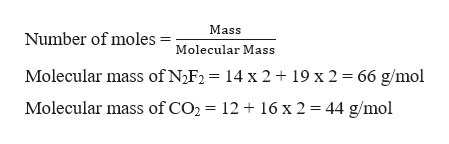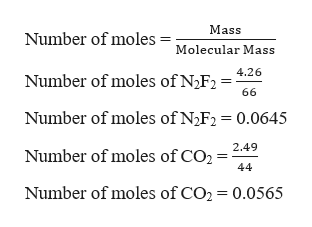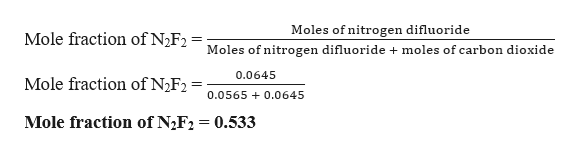# A 7.00L tank at 22.6°C is filled with 4.26g of dinitrogen difluoride gas and 2.49g of carbon dioxide gas. You can assume both gases behave as ideal gases under these conditions.Calculate the mole fraction of each gas. Be sure each of your answer entries has the correct number of significant digits.gasmole fractiondinitrogen difluoride carbon dioxide

Question
57 views

A 7.00L tank at 22.6°C is filled with 4.26g of dinitrogen difluoride gas and 2.49g of carbon dioxide gas. You can assume both gases behave as ideal gases under these conditions.

Calculate the mole fraction of each gas. Be sure each of your answer entries has the correct number of significant digits.

 gas mole fraction dinitrogen difluoride carbon dioxide

check_circle

Step 1

The mass of nitrogen difluoride is 4.26 g and that of carbon dioxide is 2.49 g. To calculate the mole fraction of each gas, it is required to calculate the number of moles of each gas,help_outlineImage TranscriptioncloseMass Number of moles Molecular Mass Molecular mass of N2F2 = 14 x 2 19 x 2 = 66 g/mol Molecular mass of CO2 1216 x 2 44 g/mol fullscreen
Step 2

Calculating the number of moles of the gases,help_outlineImage TranscriptioncloseMass Number of moles Molecular Mass 4.26 Number of moles of N2F2 66 Number of moles of N2F2 0.0645 2.49 Number of moles of CO2 44 Number of moles of CO2 = 0.0565 fullscreen
Step 3

Now calculating the mole fraction o...help_outlineImage TranscriptioncloseMoles of nitrogen difluoride Mole fraction of N2F2 Moles of nitrogen difluoride + moles of carbon dioxide 0.0645 Mole fraction of N2F2 0.0565 0.0645 Mole fraction of N2F2 0.533 fullscreen

### Want to see the full answer?

See Solution

#### Want to see this answer and more?

Solutions are written by subject experts who are available 24/7. Questions are typically answered within 1 hour.*

See Solution
*Response times may vary by subject and question.
Tagged in

### General Chemistry